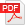Full presentation

# CFD simulation and modeling of multiphase free surface flow inside a horizontal tank for dynamic mixing of non-Newtonian food fluids

### Matteo Folezzani, Giuseppe Vignali University of Parma

The present work aims to modeling a multiphase free surface flow of non-Newtonian food fluids inside a horizontal tank. The Ostwald De Waele (Power law) equation was used to describe the shear thinning behavior of the modeled food fluids. The numerical simulations were carried out using an academic CFD version (ANSYS CFX 14.5) to perform the flow field and the free surface shape thanks to the resolution of the Reynolds averaged continuity and Navier–Stokes equations. The multiphase behavior has been modeled thanks to an Eulerian–Eulerian approach in a fully transient state. To model the free surface deformation, an homogeneous model was chosen and expressions to specify the level of free surface, density, pressure and volume fraction for each component were inserted. This model was coupled with a homogeneous k-ε turbulence model, which assumes that the turbulent quantities are the same for the two phases. The transient simulation was run with a total time of 48 seconds using a timestep of 0.25 seconds. Each time step ensure a constant agitator angle rotation of 7.5° in respect of the angular velocity of 5 rpm.